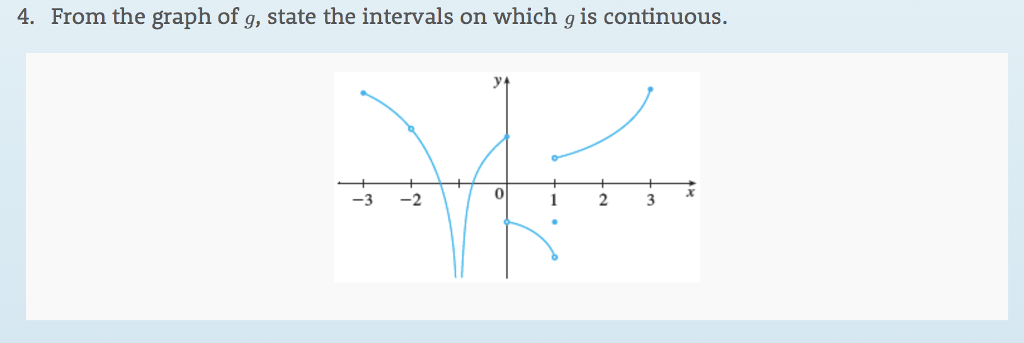# Question Solved1 AnswerFrom the graph of g, state the intervals on which g is continuous. Prom the graph of,state the intervals on which 9ils 4. From the graph of g, state the intervals on which g is continuous. 0ID6X60 The Asker · Other Mathematics

From the graph of g, state the intervals on which g is continuous.Transcribed Image Text: Prom the graph of,state the intervals on which 9ils 4. From the graph of g, state the intervals on which g is continuous. 0
More
Transcribed Image Text: Prom the graph of,state the intervals on which 9ils 4. From the graph of g, state the intervals on which g is continuous. 0